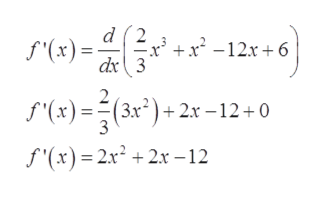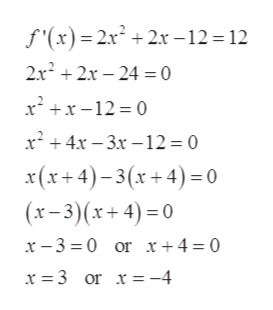Let f(x) = 2/3x3 + x2 − 12x + 6. Find the values of x for which the following are satisfied. (Enter your answers as comma-separated lists.)f'(x)=12f'(x)=0f'(x)=-12

Question

Let

f(x) = 2/3x3 + x2 − 12x + 6.

Find the values of x for which the following are satisfied. (Enter your answers as comma-separated lists.)

f'(x)=12

f'(x)=0

f'(x)=-12

Step 1

Consider the given function.

Step 2

On differentiating with respect to x, we gethelp_outlineImage Transcriptionclosed 2 +x2 -12x6 .x5 s'(x)= dx 3 S(x)=(3) Bx*)+2x -12+0 f'(x)2x2 2x-12 fullscreen
Step 3

If f’(x) =...help_outlineImage Transcriptionclosef'(x) 2x22x -12 = 12 2x22x24 = 0 xx-12 0 x24x 3x -12 0 x(x+4)-3(x+4)0 (x-3)(x+4) 0 or x+4 = 0 x-3 0 x = 3 or x = -4 fullscreen

Want to see the full answer?

See Solution

Want to see this answer and more?

Our solutions are written by experts, many with advanced degrees, and available 24/7

See Solution
Tagged in

Derivative